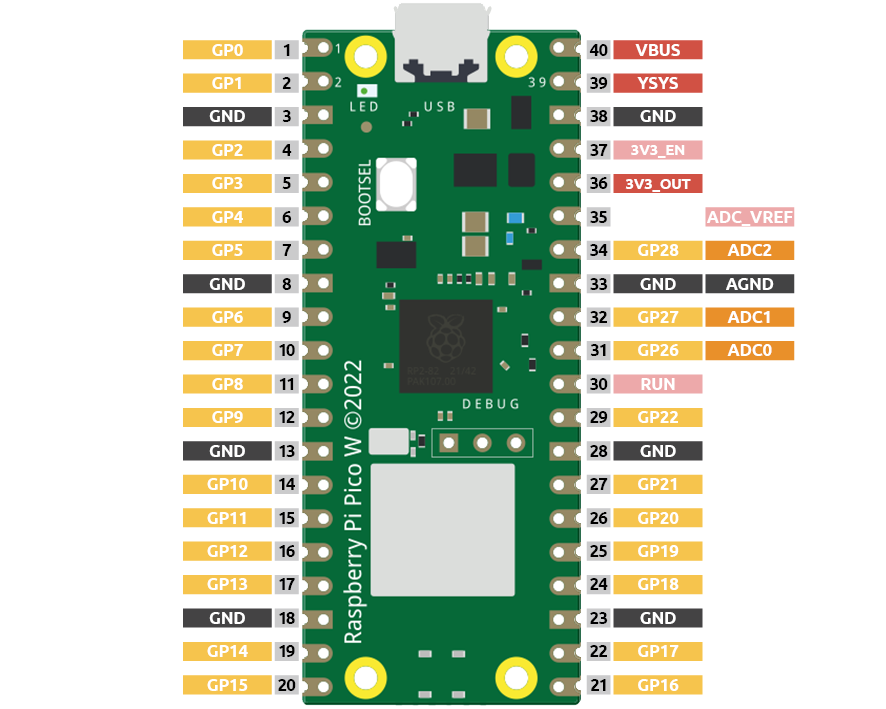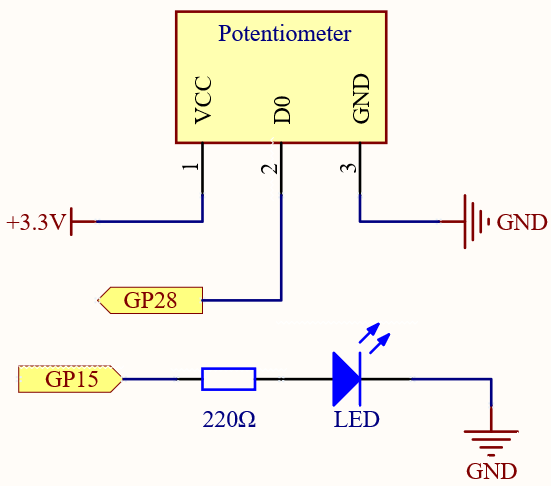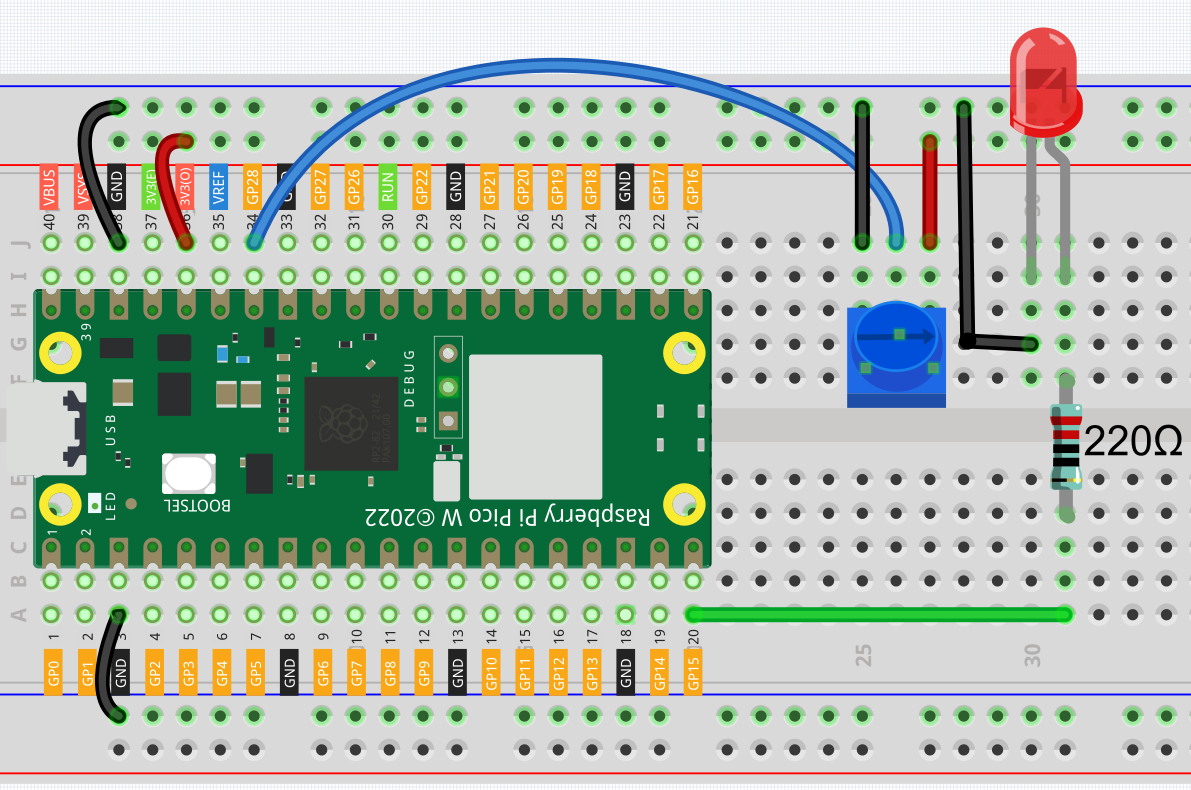# 2.11 - Turn the Knob¶

In the previous projects, we have used the digital input on the Pico W. For example, a button can change the pin from low level (off) to high level (on). This is a binary working state.

However, Pico W can receive another type of input signal: analog input. It can be in any state from fully closed to fully open, and has a range of possible values. The analog input allows the microcontroller to sense the light intensity, sound intensity, temperature, humidity, etc. of the physical world.Pico W has three GPIO pins that can use analog input, GP26, GP27, GP28. That is, analog channels 0, 1, and 2. In addition, there is a fourth analog channel, which is connected to the built-in temperature sensor and will not be introduced here.

In this project, we try to read the analog value of potentiometer.

Required Components

In this project, we need the following components.

Name

ITEMS IN THIS KIT

Kepler Kit

450+

Kepler Kit

SN

COMPONENT INTRODUCTION

QUANTITY

1

Raspberry Pi Pico W

1

2

Micro USB Cable

1

3

1

4

Jumper Wires

Several

5

Resistor

1(220Ω)

6

LED

1

7

Potentiometer

1

SchematicThe potentiometer is an analog device and when you turn it in 2 different directions.

Connect the middle pin of the potentiometer to the analog pin GP28. The Raspberry Pi Pico W contains a multi-channel, 16-bit analog-to-digital converter. This means that it maps the input voltage between 0 and the operating voltage (3.3V) to an integer value between 0 and 65535, so the GP28 value ranges from 0 to 65535.

The calculation formula is shown below.

(Vp/3.3V) x 65535 = Ap

Then program the value of GP28 (potentiometer) as the PWM value of GP15 (LED). This way you will find that by rotating the potentiometer, the brightness of the LED will change at the same time.

WiringCode

Note

• You can open the file `2.11_turn_the_knob.ino` under the path of `kepler-kit-main/arduino/2.11_turn_the_knob`.

• Or copy this code into Arduino IDE.

• Don’t forget to select the board(Raspberry Pi Pico) and the correct port before clicking the Upload button.

When the program is running, we can see the analog value currently read by the GP28 pin in the Serial monitor. Turn the knob, and the value will change from 0 to 1023. At the same time, the brightness of the LED will increase as the analog value increases.

How it works?

To enable Serial Monitor, you need to start serial communication in `setup()` and set the datarate to 9600.

```void setup() {
pinMode(ledPin, OUTPUT);
Serial.begin(9600);
}
```

In the loop function, the value of the potentiometer is read, then the value is mapped from 0-1023 to 0-255 and finally the value after the mapping is used to control the brightness of the LED.

```void loop() {
Serial.println(sensorValue);
int brightness = map(sensorValue, 0, 1023, 0, 255);
analogWrite(ledPin, brightness);
}
```
• analogRead() is used to read the value of the sensorPin (potentiometer) and assigns it to the variable `sensorValue`.

```int sensorValue = analogRead(sensorPin);
```
• Print the value of SensorValue in Serial Monitor.

```Serial.println(sensorValue);
```
• Here, the map(value, fromLow, fromHigh, toLow, toHigh) function is required as the potentiometer value read is in the range 0-1023 and the value of a PWM pin is in the range 0-255. It is used to Re-maps a number from one range to another. That is, a value of fromLow would get mapped to toLow, a value of fromHigh to toHigh, values in-between to values in-between, etc.

```int brightness = map(sensorValue, 0, 1023, 0, 255);
```
• Now we can use this value to control the brightness of the LED.

```analogWrite(ledPin,brightness);
```